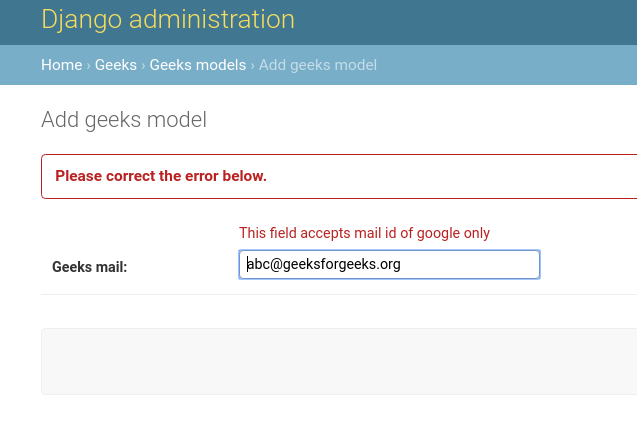# Custom Field Validations in Django Models

This article revolves around how to add custom validation to a particular field. For example to add validation of an email to a CharField by specifying a particular format. There can be multiple ways on how to achieve custom validation. In this article, we are going to show it from the model itself so that you need not manipulate it elsewhere.
These Validation are run when you are trying to create an instance of a model. Technically, this validation is implemented after you run `ModelName.objects.create(data = data)`.

Syntax –

`field_name = models.Field(validators = [function 1, function 2])`

## Django Custom Field Validation Explanation

Illustration of validators using an Example. Consider a project named `geeksforgeeks` having an app named `geeks`.

Refer to the following articles to check how to create a project and an app in Django.

Enter the following code into `models.py` file of geeks app. We will be using CharField for experimenting for all field options.

 `from` `django.db ``import` `models ` `from` `django.db.models ``import` `Model ` `# Create your models here. ` ` `  `class` `GeeksModel(Model): ` `    ``geeks_mail ``=` `models.CharField( ` `                    ``max_length ``=` `200``,   ` `                    ``) `

Now we will apply a custom validation so that the above field is validated for google mail IDs only. Create a function which accepts an argument called value. One can apply any type of operation on value now. so let us check if our function value contains @gmail.com to be validated for google mail IDs only.

 `from` `django.db ``import` `models ` `# importing validationerror ` `from` `django.core.exceptions ``import` `ValidationError ` ` `  `# creating a validator function ` `def` `validate_geeks_mail(value): ` `    ``if` `"@gmail.com"` `in` `value: ` `        ``return` `value ` `    ``else``: ` `        ``raise` `ValidationError(``"This field accepts mail id of google only"``) ` ` `  ` `  `# Create your models here. ` `class` `GeeksModel(models.Model): ` `    ``geeks_mail ``=` `models.CharField(max_length ``=` `200``) `

Now let us add this function as a validator in our field. Note that one validator function can be used for multiple fields simultaneously.

 `from` `django.db ``import` `models ` `# importing validationerror ` `from` `django.core.exceptions ``import` `ValidationError ` ` `  `# creating a validator function ` `def` `validate_geeks_mail(value): ` `    ``if` `"@gmail.com"` `in` `value: ` `        ``return` `value ` `    ``else``: ` `        ``raise` `ValidationError(``"This field accepts mail id of google only"``) ` ` `  ` `  `# Create your models here. ` `class` `GeeksModel(models.Model): ` `    ``geeks_mail ``=` `models.CharField( ` `                         ``max_length ``=` `200``, ` `                         ``validators ``=``[validate_geeks_mail] ` `                         ``) `

Let us try to create an instance without gmail.com and check if our validation worked or not. Note that after every change in models.py one needs to run makemigrations and migrate commmands.
Let us check if it gets saved in database.So the validation worked and this field can only accept email ids ending with @gmail.com. This way one can apply any kind of custom validation on `value`.

My Personal Notes arrow_drop_upCheck out this Author's contributed articles.

If you like GeeksforGeeks and would like to contribute, you can also write an article using contribute.geeksforgeeks.org or mail your article to contribute@geeksforgeeks.org. See your article appearing on the GeeksforGeeks main page and help other Geeks.

Please Improve this article if you find anything incorrect by clicking on the "Improve Article" button below.

Article Tags :

Be the First to upvote.

Please write to us at contribute@geeksforgeeks.org to report any issue with the above content.